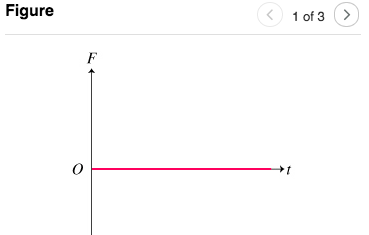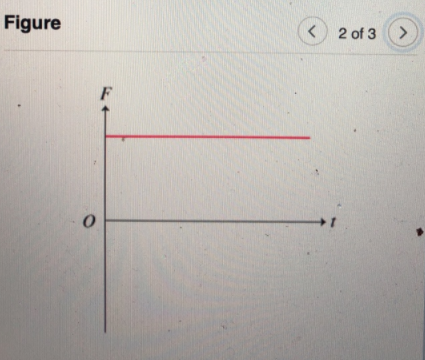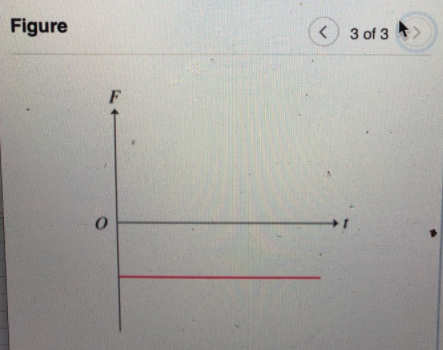# Problem: For each of the net force versus time graphs in Parts A, B, and C, construct a possible corresponding graph of velocity v(t), or position x(t), versus time. Assume one-dimensional motion.PART A: Plot velocity versus time. (Figure 1)PART B: Plot velocity versus time. (Figure 2)PART C: Plot positin versus time. (Figure 3)

###### FREE Expert Solution

In this problem, we are required to determine the effect of a constant net force on the velocity and position.

Newton's second law will be an integral part of this problem.

92% (259 ratings)###### Problem Details

For each of the net force versus time graphs in Parts A, B, and C, construct a possible corresponding graph of velocity v(t), or position x(t), versus time. Assume one-dimensional motion.PART A: Plot velocity versus time. (Figure 1)

PART B: Plot velocity versus time. (Figure 2)

PART C: Plot positin versus time. (Figure 3)